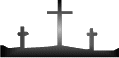```BIBLETRUTHONLINE.COM```

`  The Theory of Expounded Numbers`
```
```
``` We have talked about our theory of expounded numbers and will now
present the mathematical data and working formulas for this essential
key to the bible.

If you have not read Undersanding the Theory of Expounded Numbers,

Remember that the basis of this theory uses the same addition method
as a calculator uses: 1 + 2 = 3 ,
1 + 2 + 3 = 6,
1 + 2 + 3 + 4 = 10

FORMULA # 1:

For you mathematicians, here is one formula. . .

N2 + N
____________
2

So let's pick a number and plug it into the formula:

We'll choose 3. . .

(3)2 + (3)     9 + 3    12
_______________    = _____________  = _____  = 6
2           2       2

So, 3 expounded = 6 (CORRECT!)

FORMULA # 2:

The young mathematician, David Kavanagh, has found a new and easier
formula to use:

N x(1/2N +.5)

We'll use 3 again and plug it in. . .

3 x (1/23 +.5) = 3 x (1.5 +.5) = 3 x (2) = 6

So, 3 expounded = 6 (CORRECT!)

Now that we have presented the formulas to use and have explained
how the theory works, we will now move on to the table of expounded
numbers. (This table will purposely stop at number 77.)

The Table of Expounded Numbers

1 +

2 = 3       19 = 190      36 = 666      53 = 1431      70 = 2485

3 = 6       20 = 210      37 = 703      54 = 1485      71 = 2556

4 = 10      21 = 231      38 = 741      55 = 1540      72 = 2628

5 = 15      22 = 253      39 = 780      56 = 1596      73 = 2701

6 = 21      23 = 276      40 = 820      57 = 1653      74 = 2775

7 = 28      24 = 300      41 = 861      58 = 1711      75 = 2850

8 = 36      25 = 325      42 = 903      59 = 1770      76 = 2926

9 = 45      26 = 351      43 = 946      60 = 1830

10 = 55      27 = 378      44 = 990      61 = 1891      77 = 3003

11 = 66      28 = 406      45 = 1035     62 = 1953

12 = 78      29 = 435      46 = 1081     63 = 2016

13 = 91      30 = 465      47 = 1128     64 = 2080

14 = 105     31 = 496      48 = 1176     65 = 2145

15 = 120     32 = 528      49 = 1225     66 = 2211

16 = 136     33 = 561      50 = 1275     67 = 2278

17 = 153     34 = 595      51 = 1326     68 = 2346

18 = 171     35 = 630      52 = 1378     69 = 2415

This table is also found by clicking the link below and should be
printed for future use.

TABLE OF EXPOUNDED NUMBERS >>

Just to review, the number 9 expounded = 45,

the number 45 expounded = 1035.

So, 9 is 45 and 1035 in theory.

Back to Truth for Future Lessons >>```
```
```
`   `
` `